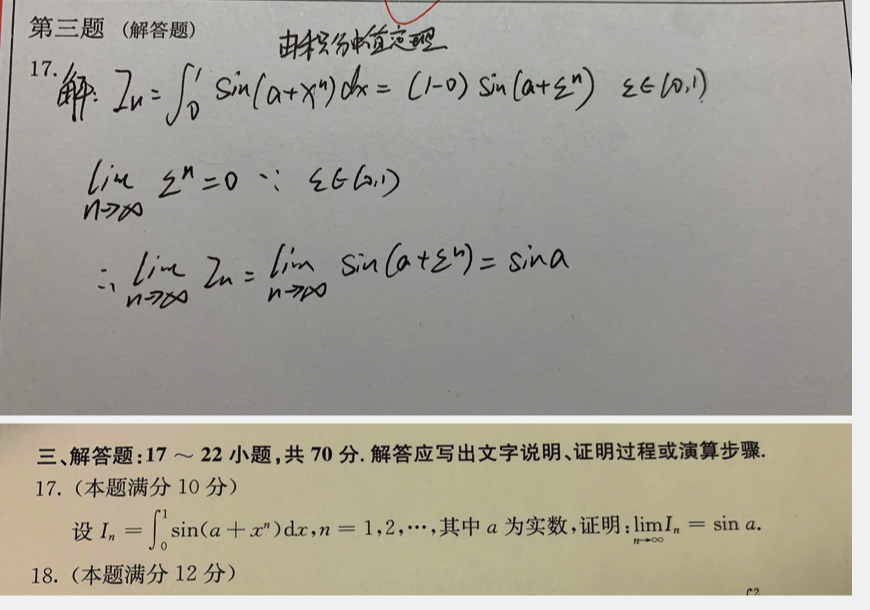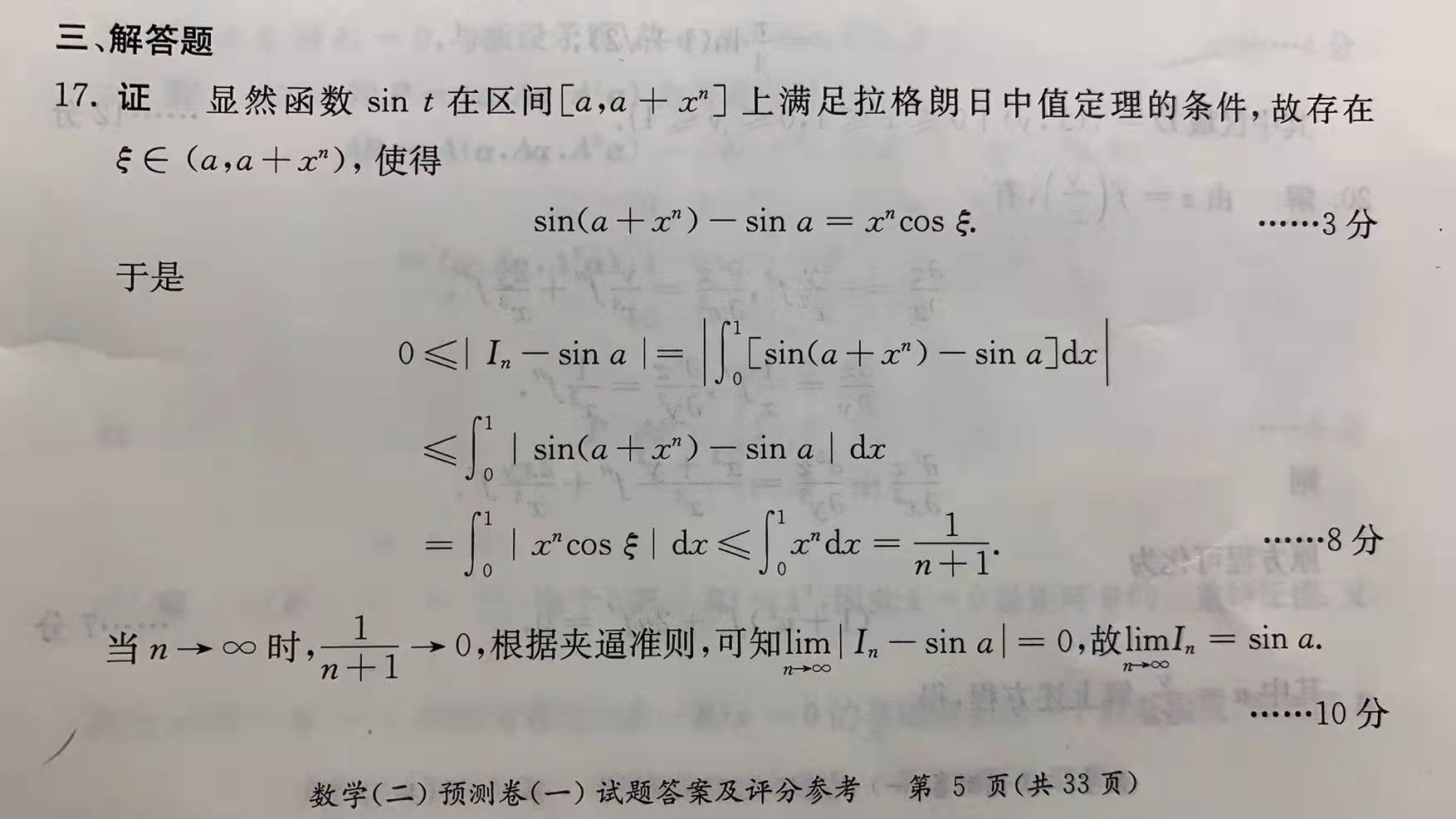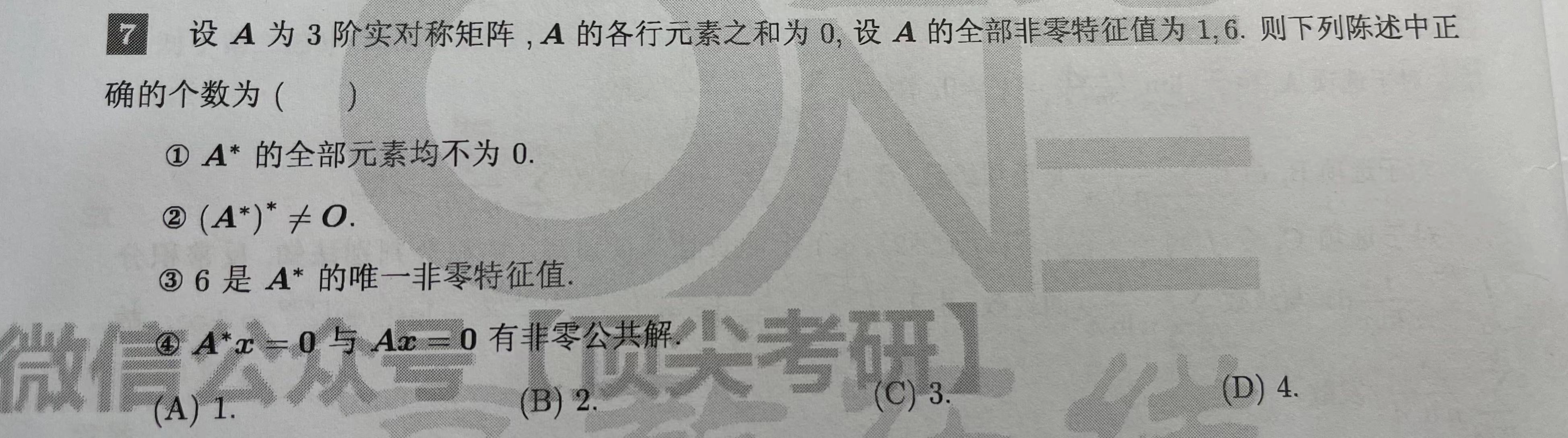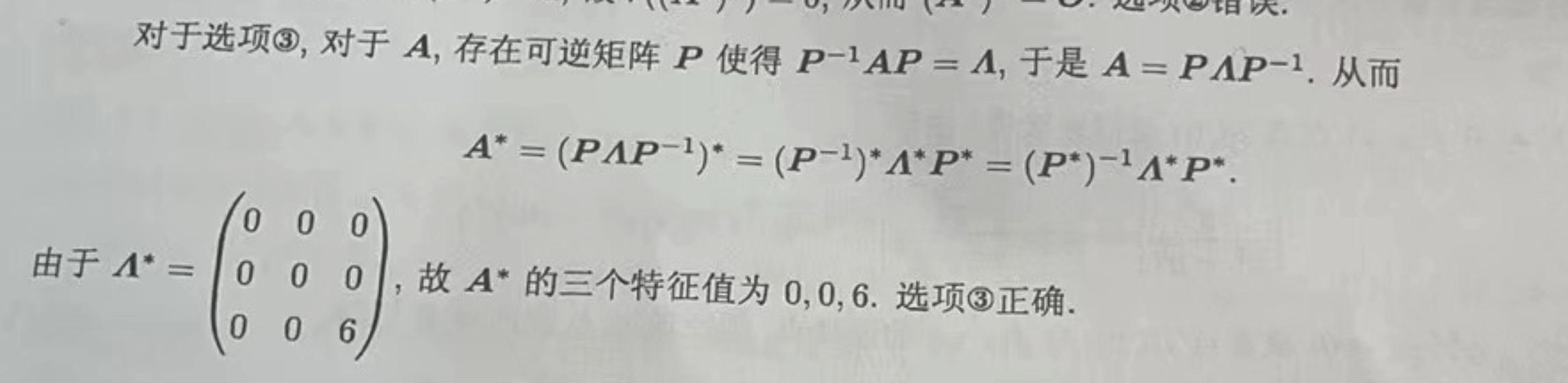# 一道“全军覆灭”的数学题

· 阅读需 5 分钟## 一道“全军覆灭”的数学题​## 该解法的错误原因​

$$\lim_{n\to\infty}{a}^n = 0, a\in(0,1)$$

$$\lim_{n\to\infty}{(1-\frac{1}{n})}^n = \frac{1}{e}\ne0$$

## 该题的正确解法​## 再举一例考研教材需要背锅的题​\begin{align} &若A可逆，其特征值为\lambda_1, \lambda_2, \lambda_3，则有：\ &A^的特征值为\frac{|A|}{\lambda_1},\frac{|A|}{\lambda_2},\frac{|A|}{\lambda_3} \end{align*}\begin{align} &对于可逆或不可逆矩阵A，其特征值分别为\lambda_1, \lambda_2, \lambda_3，\ &则有，A^的特征值为\lambda2 \lambda_3, \lambda_3\lambda_1, \lambda_1 \lambda_2，\ &而当A可逆时，则正好有\frac{|A|}{\lambda_1},\frac{|A|}{\lambda_2},\frac{|A|}{\lambda_3}，因为|A| = \lambda_1 \times \lambda_2 \times \lambda_3\ &而当A不可逆时，由于|A|=0，无法基于|A|除以各\lambda_i得到A的特征值，\ & 但\prod{j\ne i}^{n}{\lambda_j}依旧可用 \end{align*}